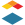# [New Quizzes] Attach multiple quiz questions to one "formula" like stimulus

Problem statement:

The formula-based questions are an essential tool for math based courses, but there is a big limiting factor. Formula questions work on a set of defined variables input by the teacher. I would like to be able to ask multiple, related formula-based questions with a shared set of defined variables.

Q1) A `m` kg ball is on Earth, what is its mass?

Q2) What is its weight?

Q3) What is its mass on the moon?

Proposed solution:

Allow for variables to be defined in quiz "stimulus". Then questions could be linked to that stimulus.

User role(s):

instructor, student